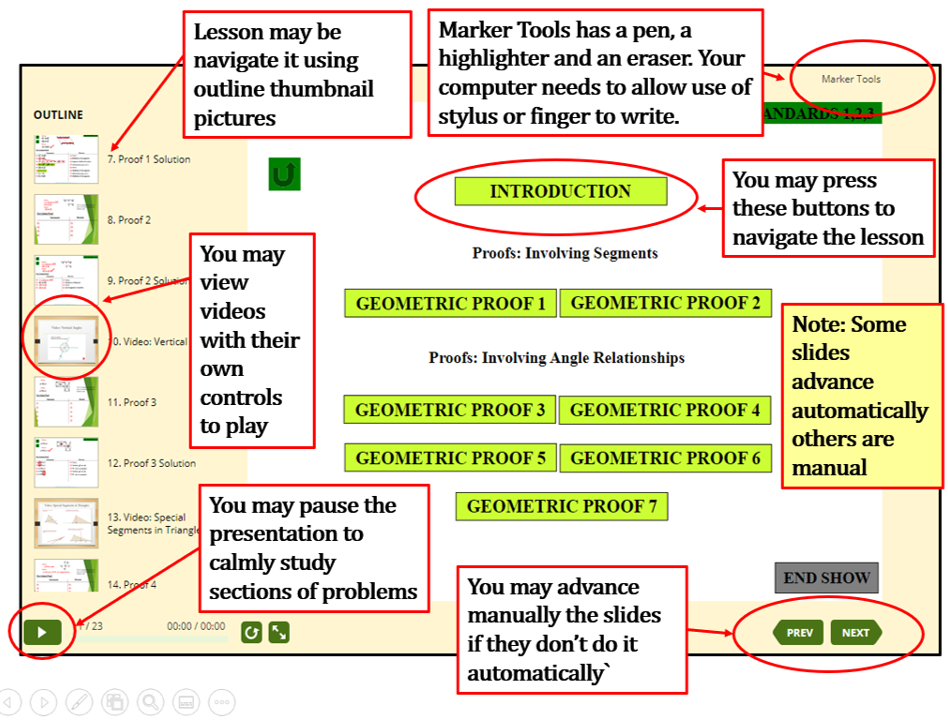QUICK SITE TOUR VIDEO OF HOW TO INTERACT

WITH THESE LESSONS (Click inside the graphic and Press Play Arrow Buttom)

# ALGEBRA 1* and 2

Lesson with an (*) are Algebra 1 Lessons. Lessons without and (*) are Algebra 2 Lessons.

scroll down to find the lessons

 CHAPTER 1 EXPRESSIONS AND FORMULAS ONE VARIABLE EQUATIONS AND INEQUALITIES 1.- Expressions and Formulas: Order of Operations.* 2.- Real Numbers: Properties of Numbers, and Number Sets.* 3.- One Variable Linear Equations Including Absolute Value.* 4.- One Variable Linear Inequalities Including Absolute Value.* (For Inequalities Real World Applications lesson.* See chapter 7) CHAPTER 2 RELATIONS AND FUNCTIONS SYSTEMS OF TWO VARIABLE EQUATIONS AND INEQUALITIES 1.- Relations and Functions.* 2.- Introduction to Slope of a Line.* 3.- Two Variable Systems of Linear Equations.* 4.- Two Variable Systems of Linear Inequalities.* CHAPTER 3 OTHER WAYS TO SOLVE SYSTEMS OF LINEAR EQUATIONS LINEAR PROGRAMING 1.- Using Determinants to Solve Systems of Linear Equations: Cramer's Rule. 2.- Using Augmented Matrix to Solve Systems of Linear Equations. Introduction of Matrices and their operations. 3.- Linear Programing: Using a Set of Coinditions to Create a Polygon and Using the Vertices to Optimize an Optimizing Function. CHAPTER 4 ARITHMATIC OF POLYNOMIALS AND PROPERTIES OF EXPONENTS RADICAL EQUATIONS AND ONE INTRODUCTION TO COMPLEX NUMBERS. 1.- Adding, Subracting, Multiplying and Dividing Polynomials 1* 2.- Adding, Subracting, Multiplying and Dividing Polynomials 2* 3.- Properties of Exponents. Adding, Subracting, Multiplying and Dividing.* 4.- Rational Exponents and Radicals. Adding, Subtracting, Multiplying and Dividing.* 5.- Radical Equations and Inequalities. Determining valid domain and solution.* 6.- Complex Numbers. Adding, Subracting, Multiplying and Dividing. CHAPTER 5 ANALITIC GEOMETRY CONICS: PARABOLA, CIRCLE, ELLIPSE, HYPERBOLE 1.- Quadratic Functions.* 2.- Distance Formula, and Midpoint Formula.* 3.- Parabola: From Graph to Equation and From Equation to Graph. 4.- Circle: From Graph to Equation and From Equation to Graph. 5.- Ellipse: From Graph to Equation and From Equation to Graph. 6.- Hyperbole: From Graph to Equation and From Equation to Graph. 7.- Conic Sections ad Theinr Generator 8.- Conic Sections and The General Equation for Conics. 9.- Inequalities with All Conics. CHAPTER 6 FUNCTIONS THAT INVOLVE POLYNOMIALS 1.- Polynomials and Zeros, Roots or Solutions (Intersections with x-axis) 2.- Mathematical Models Activity that Yields Polynomial Functions. 3.- Rational Zero Theorem 4.- Quadratic Technics to Solve Polynomial Functions of Higher Degree. 5.- Composition of Functions, and Inverse of Functions. INSIDE EACH LESSON YOU MAY DO AS THIS GRAPHIC SHOWSCHAPTER 7 REAL WORLD APPLICATIONS AND PROOFS FOR CONICS AND POLYNOMIAL FUNCTIONS AND INEQUALITIES 1.-Inequalities Real World Applications.* 2.- Ellipse Formula Proof. 3.- Hyperbole Formula Proof. 4.- Parabola Formula Proof. 5.- A Ball Up to a Ramp Generates a Parabolic Model.* 6.- Animation for the Ball Up to a Ramp (Vernier LabPro 2) 7.- Geometry and Algebra to Represent Arithmetic with Polynomials using Base 10 or Foam Prisms.* CHAPTER 8 RATIONAL EQUATIONS, EXPONENTIAL AND LOGARITMIC EQUATIONS AND AN INTRODUCTION TO SEQUENCES AND SERIES (ARITHMETIC AND GEOMETRIC) 1.- Rational Equations.* 2.- Exponential and Logarithmic Equations. 3.- Arithmetic and Geometic Sequences and Series. CHAPTER 9 PROBABILITY AND STATISTICS TOPICS (Topics in these lessons are also in the section for statistics) 1.- Measures of Central Tendency and Dispersion: Mean, Median and Mode. Standard Deviation and Variance. 2.- Counting Principle. 3- Combinations and Permutations. 4.- Experimental Probability (Introduction) 5.- Adding Probabilities (Module "OR") and Multiplying Probabilities (Module "AND") (From Informal to Formal) 6- Binomial Probability. Calculated with the formula, the tables and with Ti-83 Texas Instrument Calculator. 7- Normal Distribution: Introduction to Standard Normal Distribution and Z-score CHAPTER 10 PLAYING DICE, CARDS AND THE WHEEL OF FORTUNE. A HANDS ON APPROACH TO LEARN PROBABILITY. 1.- Real World Probability with a Spinning Wheel. (You fill in the tables) 2.- Real World Probability with a Spinning Wheel (Tables already fill) 3.- Real World Probability with Dice and Cards (You fill the tables) 3.- Real World Probability with Dice and Cards (Tables already fill) CHAPTER 11 ADVANCED TOPICS OF TRIGONOMETRY 7.- Trigonometry An In Depth Approach to Sine, Cosine, Tangent, Cotangent, Secant and Cosecant: Trigonometry Ratios and Their Graphs and Real World Applications. 8.- Trigonometry Identities. Sum and Difference, Double Angle and Half Angle. PROOFS

HOME

LONG DURATION DEMO OF HOW TO INTERACT (Several Minutes but worthwhile)

 Algebra 1 and 2 Geometry Basic Math Statistics and Probability Quizzes for Geometry